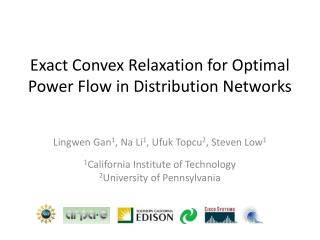DownloadDownload PresentationExact Convex R elaxation for Optimal Power Flow in Distribution Networks

# Exact Convex R elaxation for Optimal Power Flow in Distribution Networks

Télécharger la présentation## Exact Convex R elaxation for Optimal Power Flow in Distribution Networks

- - - - - - - - - - - - - - - - - - - - - - - - - - - E N D - - - - - - - - - - - - - - - - - - - - - - - - - - -
##### Presentation Transcript

1. Exact Convex Relaxation for Optimal Power Flow in Distribution Networks Lingwen Gan1, Na Li1, Ufuk Topcu2, Steven Low1 1California Institute of Technology 2University of Pennsylvania

2. Optimal power flow indistribution networks • Optimal power flow (OPF) has been studied in transmission networks for over 50 years • DC (linear) approximation • Voltage close to nominal value • small power loss • small voltage angle • Volt/VAR control, demand response problems motivate OPF in distribution (tree) networks • Have to solve nonlinear, nonconvexpower flow No longer true in distribution networks

3. How to solve nonconvex power flow? • (Heuristic) nonconvex programming. • Hard to guarantee optimality. • Convexify the problem. • Relax the feasible set A to its convex hull. • If solution is in A, then done. • In general, don’t know when relaxation is exact. A Def: If every solution lies in A, then call the relaxation exact.

4. Outline • Formulate OPF. • Convex relaxation is in general not exact. • Propose a modified OPF. • Modified OPF has an exact convex relaxation.

5. An OPF example • Volt/VAR control • Potential controllable elements • Inverters of PV panels • Controllable loads (shunt capacitors, EVs)

6. Mathematical formulation For simplicity, let’s look at one-line networks. Bus 0 Bus 1 Bus n

7. Mathematical formulation Bus 0 Bus 1 Bus n f is strictly increasing in power loss Nonconvex

8. Convex relaxation OPF SOCP A Q: does every solution to SOCP lie in A?

9. Is SOCP exact? In general, no. 1 unit power generation, p injected into the grid, 1-p gets curtailed.

10. What do we do when non-exact? Modify OPF in order to obtain an exact convex relaxation. A A’ B’ • We want • the grey area to be small; • the relaxation to be exact after modification.

11. The modification OPF OPF-m What is vilin(p,q)?

12. What is vilin(p,q)? A’ • An affine function of p and q. • is a linear constraint on p and q. • An upper bound on vi. • Smaller feasible set than OPF.

13. Grey area is empirically small and “bad” is a good approximation of v Grey area is small. Grey area is “bad”. A’ w

14. Convex relaxation OPF-m SOCP-m A’ Q: Does every solution to SOCP-m lie in A’?

15. Exactness of SOCP-m Thm: If condition (*) holds, then the SOCP-m relaxation is exact; the SOCP-m has a unique solution. • Condition (*) • can be checked prior to solving SOCP-m; • holds for all test networks (see later); • imposes “small” distributed generation.

16. Condition (*) • Depend only on parameters , not solutions of OPF-m or SOCP-m. • Impose small distributed generation.

17. How to check (*)? much stricter than (*)!

18. (*) holds with significant margin IEEE network: no distributed generation. Worst case: maximizes . No load, all capacitors are switched on.

19. (*) holds with significant margin SCE network: 5 PVs with 6.4MW nameplate generation capacity (11.3MW peak load). Worst case: maximizes . No load, all PVs are generating at full capacity, all capacitors are switched on.

20. Summary • The SOCP relaxation for OPF is in general not exact • Propose OPF-m • The convex relaxation SOCP-m is exact if (*) holds • (*) widely holds in test networks Thank you!